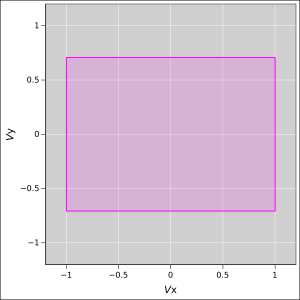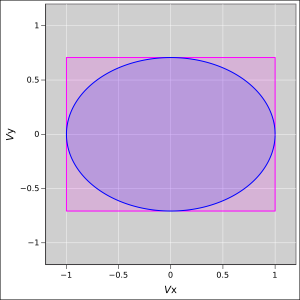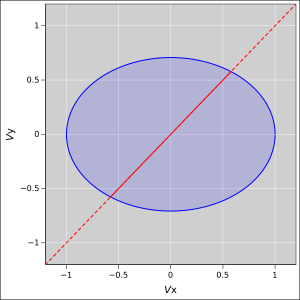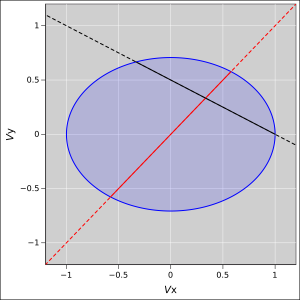[Contents]

Modeling a Collision
with Successive Refinement

John Denker

## 1  Building and Refining the Model

Suppose we have two carts, and we want to build a model to describe what happens when they collide.

• The first cart has one unit of mass, and initially has Vx = 1 in some units, relative to the lab frame.
• The second card has two units of mass, and is initially at rest in the lab frame.

We assume there are no hidden reservoirs of potential energy, i.e. no motors and no jack-in-the-box mechanisms that will spring out during the collision. Similarly we assume the track is level, so we don’t need to worry about gravitational potential energy.

We also assume there is no transfer of momentum from the carts to the surroundings. We further assume the motion is confined to one dimension.

Using the simplest notions of energy, we can build the model shown in figure 1, which puts bounds on the final Vx and Vy. The first cart cannot be going faster than 1 unit, because that would require more energy than we have. Similarly the second cart cannot be going faster than 1/√2, because that would require more energy than we have.Figure 1: Bound on Each Energy

We now refine the model. The bounds on the energy as shown in figure 1 are not wrong, but we can do better. Conservation of energy puts a bound on the total energy, not just the individual energies. This is shown by the ellipse in figure 2.

All possible elastic collisions result in (Vx, Vy) values that sit on the ellipse. Inelastic collisions sit in the interior.Figure 2: Bound on Total Energy

We can make the model more elaborate. In particular, the two carts may or may not stick together after the collision. If they do, then the final velocities will lie along the red line in figure 3.Figure 3: Maybe They Stick Together

The dotted portion of the red line satisfies the requirement that the two carts are stuck together, but is infeasible for energy reasons.

The rectangular region is not shown in figure 3, because it is no longer needed. For discussion of this point, see section 3.1.

We now make a further refinement to the model. We make use of the fact that momentum must be conserved. Any final (Vx, Vy) values that conserve momentum lie along the black line in figure 4. All other values are forbidden for momentum reasons.Figure 4: Conservation of Momentum

## 2  Remarks about the Physics

At this level of detail, any point along the solid black line is a feasible outcome. Points near the right end correspond to carts brushing past each other and barely interacting. Points near the left end correspond to an elastic collision, where the first cart bounces cleanly off the second cart. Points in the interior correspond to various degrees of stickiness during the interaction.

One thing we see immediately is that the red line and the black line intersect deep in the interior of the ellipse. Therefore we know that if the two carts stick together, the interaction cannot possibly be elastic. The mechanism that holds them together must include some sort of damper, some sort of shock absorber that converts macroscopic kinetic energy to something else.

In the real world, the couplers (draft gears) on rail cars do in fact include a damper, for exactly this reason.

Another thing we see is that it is not possible for the first cart to transfer all its energy to the second cart. In the most favorable case, i.e. an elastic collision, the first cart bounces off the second cart and is left with a backwards Vx component, which involves some nonzero kinetic energy.

(In a different scenario, under special conditions it is possible – ideally – to transfer 100% of the energy, but this requires the two carts to have identical masses.)

Question: Did you notice that the red line bisects the solid black line? Can you explain this? Is it related to the 2-to-1 mass ratio? What would happen for other mass ratios?

## 3  Remarks about the Process

### 3.1  Succesive Refinement

The process described here is an example of successive refinement. That is, we start with a relatively crude model, because we have to start somewhere. Then we make it more nuanced and more elaborate, step by step, by bringing in additional ideas.

The rectangular region that appears in the early versions of the model does not appear in figure 3 and later versions. That’s because it doesn’t tell us anything that the ellipse isn’t already telling us. This illustrates an important point: Sometimes refinement replaces or removes features from the model. It is not necessarily a process of monotonic accumulation. A hard-to-use approximation may be replaced by a more convenient one. A loose approximation may be replaced by a tighter one.

Note that if a certain line of inquiry turns out to be a dead end, that does not mean it was a mistake. When you’re running a maze, knowing where the dead ends are is valuable information. For more discussion of this point, including an interactive maze, see reference 1.

The idea of successive refinement applies very broadly. Examples include theoretical physics, engineering, writing computer programs, writing a novel, composing music, et cetera.

If you cannot solve the assigned problem all at once, sometimes it helps to start by working on a similar but simpler problem. Think of it as a warm-up exercise. The opposite idea is also good advice: After solving the assigned problem, go back and see if the same ideas can be used to solve a broader class of problems. For more about thinking in general, and warm-up exercises in particular, see reference 2, reference 3, and reference 4.

### 3.2  Controlled Approximation

It must be emphasized that a model does not need to make an exact prediction to be useful. Often, for planning purposes, it suffices to be able to bracket the possible outcomes. For example, when rolling a pair of dice, we can say that the outcome will be between 2 and 12 inclusive, even though we cannot predict the outcome exactly.

So it is with our collision model in figure 4: At this level of detail, we know the outcome will sit somewhere along the solid black line. To determine the outcome more exactly would require additional information about the interaction.

Our collision model does not make a unique specific prediction, but even so, it has some value. For one thing, it brackets the possible outcomes. This helps when planning the experiment, for safety if nothing else. Also, it tells us what sort of additional information is needed.

## 4  References

1.
John Denker,
“How Research is Done : Exploring a Maze using only Local Information”
www.av8n.com/physics/research-maze.htm

2.
John Denker,
“Learning, Remembering, and Thinking”
www.av8n.com/physics/thinking.htm

3.
John Denker
“Problem-Solving Checklist”
www.av8n.com/physics/problem-solving-checklist.htm

4.
George Pólya,
How to Solve It: A New Aspect of Mathematical Method
1st edition (1945), 2nd edition (1957), Princeton University Press
[Contents]